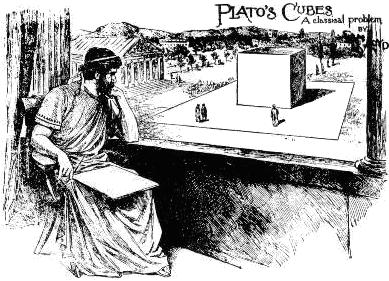## Can you better Sam Loyd?

Those in search of a next mathematical project may want to look through the treasure trove of puzzles bequeathed by Sam Loyd, the greatest American puzzlist. Born in 1841, he invented (according to his own account) his first puzzle at the age of 9. When he was 16 he became problem editor of the Chess Monthly and made a good living of puzzle invention till his death in 1911. One of his problems (Fifteen) caused a national craze on both sides of the Atlantic. Some other puzzles also sold by the millions. Loyd's ingenuity might only be compared to that of his contemporary, H.E. Dudeney - England's greatest puzzlist.

The project may consist in skimming through Loyd's puzzles with a bold idea of coming up with a solution to one of the puzzles that is different from or even better in some sense than Loyd's original solution. The task may not be all that difficult because his audience of a century ago very much shared in the modern attitude towards mathematics. His geometric puzzles often require true ingenuity, but those that call for numerical manipulations can, in most cases, be solved by simple algebraic methods. Loyd's solutions are mostly arithmetic.

Here's one example (Mathematical Puzzles of Sam Loyd selected and edited by Martin Gardner (Dover, 1959), #71, p. 68).The sketch shows Plato gazing upon the huge marble cube which is constructed out of a given number of smaller cubes. The monument rests in the center of a square plaza, paved with similar small cubic blocks of marble. There are just as many cubes in the pavement as there are in the monument, and they are precisely of the same size. Tell how many cubes are required to construct the monument and the square plaza upon which it stands, and you will have solved the great problem of Plato's Geometrical Numbers.

### Sam Loyd's Solution

The problem calls for a number which when cubed will give a square number. This happens to be the case with any number which is itself a square. The smallest square (aside from 1) is 4, so the monument might consist of 64 small cubes (4×4×4) which would stand on the center of an 8×8 square. This, however, will not fit the proportions shown in the sketch.

We therefore try the next highest square, 9, which gives us a monument of 729 cubes standing on a 27×27 square. This is the correct answer for it is the only one which agrees with the illustration.

So, what do you say? Is there a different approach?

### Reference

1. M. Gardner, Hexaflexagons And Other Mathematical Diversions, The University of Chicago Press, 1988### Other Puzzles by Sam Loyd### Another solution

We may take a clue from Loyd's remark concerning the proportions shown in the sketch. The side of the cube appears to be 1/3 of the side of the square plaza. We may imagine the plaza cut into 9 smaller squares each of the size of the cube's base. Stacked on top of each other these nine squares must fill up the cube because the cube and the plaza consist of the same number of small cubes. It then follows that there are 9 small cubes on a side of the cube, to the total of 93.

### And another one

Sam Shah has thought up another direct solution that avoids Loyd's trial-and-error. Take a single cube. If you put same size square all around you require 9 squares (although you are using cubes you are only using top surfaces for the base, hence squares).

Straight away you know your answer has to be a multiple of 9. Now 9 * 9 * 9 = 729 hence you answer.